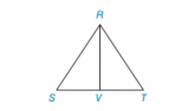Chapter 2.4, Problem 30EElementary Geometry For College St...

7th Edition
Alexander + 2 others
ISBN: 9781337614085

Solutions

Chapter
SectionElementary Geometry For College St...

7th Edition
Alexander + 2 others
ISBN: 9781337614085
Textbook Problem

Given: Equiangular Δ R S T Prove: R V → bisects ∠ S R T Δ R V S is a right ΔTo determine

To prove:

ΔRVS is a right angle triangle or not.

Explanation

Given:

Equiangular ΔRST and RV¯ bisects ΔSRT.

Figure (1)

Approach:

The sum of interior angles of a triangle is equal to 180°.

Calculation:

mS+mT+mSRT=180°

mS+mSRV+mRVS=180°

Since, bisects SRT. Therefore,

mSRV=12(mSRT)

Since, ΔRST is an equiangular triangle. Therefore,

mS=mT

Consider, mS+mSRV

Substitute 12(mSRT) for mSRV, mT for mS, and 12(2mS) for mT.

Still sussing out bartleby?

Check out a sample textbook solution.

See a sample solution

The Solution to Your Study Problems

Bartleby provides explanations to thousands of textbook problems written by our experts, many with advanced degrees!

Get Started

Evaluate the integral. 29. 33x1+xdx

Calculus: Early Transcendentals

For any events A and B with P(B) 0, show that P(A|B) + P(A|B) = 1.

Probability and Statistics for Engineering and the Sciences

Sketch the graphs of the equations in Exercises 512. xy=4

Finite Mathematics and Applied Calculus (MindTap Course List)

Find the limit or show that it does not exist. limx(x2+1)

Single Variable Calculus: Early Transcendentals, Volume I

Prove the identity cos2x=1tan2x1+tan2x.

Trigonometry (MindTap Course List)

0 1 π It does not exist.

Study Guide for Stewart's Multivariable Calculus, 8th